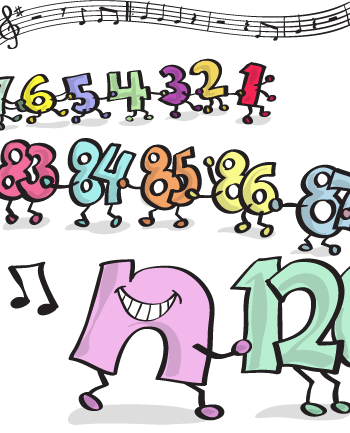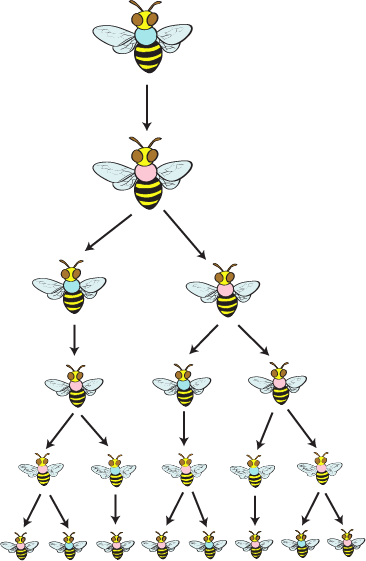## Sequences

Just like the different lists we threw out in the introduction, there are such things as mathematical lists, too. A sequence is just a list of numbers separated by commas. Like this:

1, 3, 5, 7, 9, …

This is nothing more than a sequence of odd numbers. These lists can be finite (stop at a certain point) or head to infinity and beyond. What makes these lists a little more interesting is that they can be described using things we call rules. We know—it's not a very original name.### Basic Nules and Rotation…We Mean Rules and Notation

First things first. Before we go writing rules for sequences willy-nilly, we need to establish a common notation, or way of writing these things down. It is handy to know that we'll typically start with the first term, which we'll call a1. The next term will be called a2 and then, you guessed it, a3. We can have any number of terms.

Eventually, somewhere way down the number line, we will get to some random term we'll call the nth term, "n" being the shorthand way of writing "any number." We call this term an. Go figure. We can then put an in braces, like so: {an}. This tells the reader that we're now talking about the rule that will give us the nth term. In other words, if we put 3 in for n in our rule, we would be looking at the third term in the sequence. Let's try a few.

### Sample Problem

Find the rule and a6 for the sequence: 4, 7, 10, 13, …

There is no 100%, no-doubt, sure-fire, going-to-work-every-time method to finding the rule for a sequence. Sorry. You just need to be a good problem solver and apply what you know about different operations to the numbers you're given.

Here, the first thing we might notice is that the numbers go up by 3 every time. That means that 13 + 3 = 16 and then 16 + 3 = 19. So a6 = 19. That's our sixth term.

For now, we want to put together a general rule that gives us any term. We need something that looks like {an} = ________ and uses 'n' in the actual rule, also known as an explicit rule. We're guessing we'll also need to use the number 3 somehow. Adding 3 might work, but then we have to use previous terms and we're not quite there yet. That's called a recursive rule…we'll get there later.

So what if we relied on the challenging idea of multiplication instead? Our first term (meaning n = 1) is really 1 + 3. The second is 1 + 3 + 3. The third is 1 + 3 + 3 + 3. We can conclude that the term number is the same as the number of 3s we used. That means our rule would be:

{an} = 1 + 3n

We like these rules because we can always check and make sure they work. For instance, if we plug in 6 for n to get the sixth term, we get:

a6 = 1 + 3(6) = 19

Done and done.

### Sample Problem

Find the rule and a10 for the sequence 2, 4, 8, 16, …

This one forces us to step up our game a little bit. We don't add the same number over and over this time. Instead, we add 2, then 4, then 8. But wait; wouldn't you agree that each number is just multiplied by another 2? In other words, the first term is 2, and then the second is 2 × 2, and then 2 × 2 × 2, and then 2 × 2 × 2 × 2, and then and then and then…this calls for an exponent. Therefore, our rule could be:

{an} = 2n

Let's check it. If we go back and put in 3 for n, we get:

a3 = 23 = 8

Nice. That's our third term all right, so our rule works.

We can then go back and use our rule to get a10.

a10 = 210 = 1024

### Sample Problem

Find the rule and a7 for the sequence 1, 2, 6, 24, 120, …

This problem is tough. No doubt about it. We made it that way on purpose. Shmoop is actually a league of evil educators whose sole purpose is to make your life less fun. True story.

Regardless, after taking some time to analyze the problem, you might be able to see that we multiply 1 × 2 to get 2, 2 × 3 to get 6, 2 × 3 × 4 to get 24, and so forth. So we have this idea that we need to multiply by the next biggest number in order to jump from one term to the next. This is a pretty unique math concept. In fact, we've seen it before.

It's called a factorial, and they come up a lot when talking about sequences. Math freakazoids love this stuff. Factorials are so cool, they are written with a '!', literally:

3! = 3 × 2 × 1 = 6

5! = 5 × 4 × 3 × 2 × 1 = 120.

1! is the boring number 1,  but 0! is also the number 1. Why? Ask Algebra 1.

Back to the problem at hand, where we still need a stinkin' rule for 1, 2, 6, 24, 120, …. It appears as though the first term is 1!, the second is 2!, the third is 3!, and so on. How about we make our rule for an:

{an} = n!

You can check it and see that it works with 4! and 5! as well and can now help us to find a7. This is going to be nothing more (or less) than a7 = 7! = 5040.

• ### Recursive Rules

Recursive. That word makes us think of second grade when teachers forced us to write with the cursive m that had three humps instead of two. Whoever came up with that? Either way, recursive rules have nothing to do with cursive handwriting. In fact, "recursive" is an adjective that describes a certain type of rule. It comes from the word "recursion," which is fancy lingo for something that's generated from prior or preceding terms.In plain English, a recursive rule is when we find the next term of a sequence using the one (or ones) before it. No. Big. Deal. It can actually be easier sometimes.

To figure this out, we're going to go back to the sequences we already created rules for. This time, we'll make recursive rules instead of explicit ones.

### Sample Problem

Find the recursive rule and a6 for the sequence: 4, 7, 10, 13, …

This time, we want to find a rule that uses an − 1 to get an. This is nothing more than the mathematically cool way of saying we want to use the second term to get the third, or the fifth term to get the sixth.

With this sequence, we take each term and add 3 to it. So we'll take the term that we want to know, an , and write it in terms of an − 1, or the term that came right before it.

{an} = (an – 1) + 3

The biggest downfall of recursive rules is that in order to find another term, like a6 in this case, we need to first find a5 and a4 and so on down the line. There's no plugging in n like we did before.

In this example, a4 = 13, so a5 = 13 + 3 = 16 and a6 = 16 + 3 = 19. So a6 = 19.

Not too bad. But what if we were asked for a30? Holy terms, Batman.

### Sample Problem

Find the recursive rule and a10 for the sequence 2, 4, 8, 16, …

Each term is multiplied by 2 to get the next term. This sets up B-E-A-utifully. Our rule couldn't be much finer:

{an} = 2(an − 1)

The problem we do run into is finding a10 for this guy. We only have four terms, so we'll have to do some serious multiplication. Here it goes…

a5 = 2(a4 ) = 2(16) = 32
a6 = 2(a5 ) = 2(32) = 64
a7 = 2(a6 ) = 2(64) = 128
a8 = 2(a7 ) = 2(128) = 256
a9 = 2(a8 ) = 2(256) = 512
a10 = 2(a9 ) = 2(512) = 1024

So a10 = 1024, just like it did the first time.

### Sample Problem

Find the recursive rule for the sequence 0, 1, 1, 2, 3, 5, 8, 13, …

This sequence is very famous and is known as the Fibonacci sequence. Without going into too much detail, this sequence is found all over the place, literally, and is really awesome. But don't take our word for it; check out this video. (You're going to want to watch this one.)

Now let's answer the question. We wanna find a recursive rule. This time, there is no common number added, subtracted, multiplied, or divided. There are no exponents involved. And, try as we might, there are no factorials. Instead, starting with the third term, each term in the Fibonacci Sequence is based solely on the previous two terms.

a1 = 0
a2 = 1
a3 = a2 + a1 = 1 + 0 = 1
a4 = a3 + a2 = 1 + 1 = 2
a5 = a4 + a3 = 2 + 1 = 3

And so on.

The rule must take into account both an − 1 and an − 2 which gives us:

{an} = (an – 1) + (an – 2)

Congratulations. You have officially graduated into the realm of the mathematically informed. More importantly, you now understand why this joke is funny:

A math professor walks into a Fibonacci conference, looks at an esteemed math professor colleague and says, "Man, I can't wait. I think this year's conference is going to be as big as the last two years combined."

### Bonus Problem

Fibonacci's Honeybees

In a beehive there are male and female bees. There's a catch, though. Female bees are born when a male bee fertilizes a female egg. Male bees are born when a female egg is left unfertilized. This means males have a mother but no father. Weird. Your mission: draw the first six levels of the family tree of a male honeybee.

The first level has just one male, the one whose tree we are drawing. The second level has just one bee, the male's mom. The third level has a male and female since each female has a mom and a dad. The next level will have a male and female pair in addition to another female—and so on and so fourth.Check it out. The levels of the honeybee family tree are 1, 1, 2, 3, 5, 8, … #iKnowRight